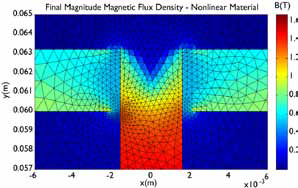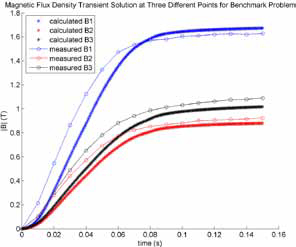Christine Ross with Advisor P.T. KreinFigure 3: Cross-section magnetic flux denisty magnitude steady-state solution for a two-demensional, MATLAB FE time-domain simulation of the benchmark problem

The research goal is to explore the acceleration potential of incorporating graphic-processing units (GPUs) for induction machine (IM) numerical field analysis. Characteristics of IM electromagnetic behavior essential to accurately simulate IMs have been studied. Using MATLAB’s Parallel Computing Toolbox, a two-dimensional finite element (FE) time-domain simulation of a benchmark problem that includes eddy current and magnetic saturation has been developed and matches the measured results well. The computational aspect of FE analysis benefits from parallel computation, as the problem involves solving a large system of equations (typically at least 2,000) and can be decomposed into sub-problems that can be solved at the same time.Figure 4: Measured and calculated magnetic flux density magnitudes at three points for the benchmark problem

Figure 3 shows the computed steadystate magnetic flux density magnitude near the center and channel steel plates. Figure 4 compares the measured and calculated magnetic flux densities at three points of the simulated structure. Parts of the program that benefit from parallel computation have been written to use GPUs, and the program is being tuned to obtain the best performance possible while still using MATLAB’s GPU computation capabilities. The same program will be used to simulate a two-dimensional model of a linear induction machine to compute the steady-state force. The computed results will be compared to measurements.
This work is funded by the Grainger Center for Electric Machinery and Electromechanics and the University of Illinois SURGE Fellowship.